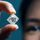cancel
Showing results for
Did you mean:Helper III

## Get the latest & most repeated Unit Prices

Hi All, I am looking to extract latest value and most repeated value (if tie get max).

Item UOM Unit Price Order Date
Item1 EA 95.00 25-Jan-22
Item1 Carton 1,800.00 24-Feb-22
Item1 EA 95.00 19-Feb-22
Item1 EA 80.00 1-Mar-22

Result should be like this:

Item UOM Latest Unit Price Most Repeated
Item1 EA 80 95

Looking for dax meaure not calculated column measure.

1 ACCEPTED SOLUTIONSolution Sage

@shareezsaleem try by creating below Measures

`UOM  = var T1 = SUMMARIZE('Table','Table'[UOM],"Count",COUNTROWS('Table'))       var T2 = MAXX(T1,[Count])     return       MAXX( T1, IF([Count] = T2,'Table'[UOM])) `

`Latest Unit Price = var _M = MAXX('Table','Table'[Order Date])                       return                            CALCULATE(MAX('Table'[Unit Price]),'Table'[Order Date] = _M)`

`Most Repeated = var T1 = SUMMARIZE('Table','Table'[Unit Price],"Count",COUNTROWS('Table'))                var T2 = MAXX(T1,[Count])             return                MAXX( T1, IF([Count] = T2,'Table'[Unit Price]))`Solution Sage

@shareezsaleem try by creating below Measures

`UOM  = var T1 = SUMMARIZE('Table','Table'[UOM],"Count",COUNTROWS('Table'))       var T2 = MAXX(T1,[Count])     return       MAXX( T1, IF([Count] = T2,'Table'[UOM])) `

`Latest Unit Price = var _M = MAXX('Table','Table'[Order Date])                       return                            CALCULATE(MAX('Table'[Unit Price]),'Table'[Order Date] = _M)`

`Most Repeated = var T1 = SUMMARIZE('Table','Table'[Unit Price],"Count",COUNTROWS('Table'))                var T2 = MAXX(T1,[Count])             return                MAXX( T1, IF([Count] = T2,'Table'[Unit Price]))`